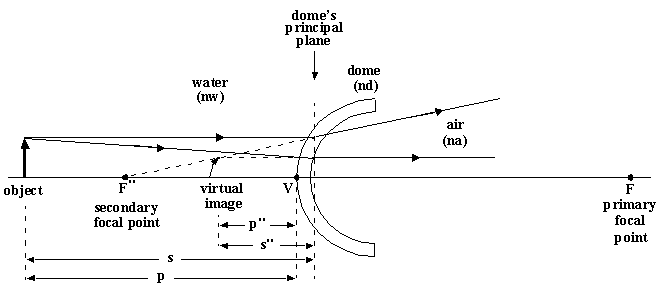# Optics of Dome Ports

The main drawback of a flat port on an underwater camera housing is the narrowed field of view caused by refraction at the planar air/water interface. The object seems closer, so that less of it is seen by the camera lens. In fact, the theoretical maximum angle of view behind a flat port is 97.2 degrees. A spherical dome port removes this problem and thus enables the underwater photographer to use wide angle lenses more effectively. In addition, it allows for very close subject distances, which decreases the in-water light path and thereby improves image brightness and color saturation.

To appreciate how a dome lens accomplishes this it is necessary to apply the principles of geometrical optics for thick lenses. The analysis is messy and the resulting equations seem complicated, but they can be used rather easily to study what actually happens. Consider the figure below, which shows a left-to-right arrangement of water/dome/air with corresponding indices of refraction nw/nd/na. We assume a concentric hemispherical dome with external surface (on the left) of radius r1, internal surface of radius r2, and dome thickness d=r1-r2.For an object to the left of the dome, the following Fortran code will compute the position and size of the image produced by the dome. The necessary inputs are nw, nd, na, r1, r2, d, and the object distance p as shown in the figure. Our assumption that d=r1-r2 (concentric dome surfaces) produces coincident primary and secondary principal planes.

```
(all variables declared real)

f1=nw*(r1/(nd-nw))      !primary focal length of dome's external surface
f1p=f1*nd/nw            !secondary focal length of dome's external surface
f2p=nd*(r2/(na-nd))     !primary focal length of dome's internal surface
f2pp=f2p*na/nd          !secondary focal length of dome's internal surface
enovrf=nd/f1p + na/f2pp - (d/f1p)*(na/f2pp)
f=nw/enovrf             !primary focal length of the dome
fpp=f*na/nw             !secondary focal length of the dome
a1f=-f*(1.-d/f2p)       !position of the dome's primary focal plane relative
!to the dome's external vertex (on the dome axis)
a2fpp=fpp*(1.-d/f1p)    !position of the dome's secondary focal plane relative
!to the dome's internal vertex (on the dome axis)
a1h=f*d/f2p             !position of the dome's primary principal plane relative
!to the dome's external vertex (on the dome axis)
a2hpp=-fpp*d/f1p        !position of the dome's secondary principal plane relative
!to the dome's internal vertex (on the dome axis)
s=p+a1h                 !p=object distance relative to external vertex
!s=object distance relative to primary principal plane
spp=na/(nw/f - nw/s)    !image distance relative to secondary principal plane
ppp=spp+a2hpp+d         !image distance relative to external vertex
m=-(nw/na)*(spp/s)      !size of image relative to size of object
view=-(spp-g)/(s+g)/m
!g=distance from dome principal plane to lens primary
!  principal plane
!view=tangent of lens' effective half-angle of view
!     divided by tangent of lens' in-air half-angle
!     of view

(These equations assume paraxial rays, i.e. rays making small angles with the dome axis). ```

If you substitute some reasonable values for the indices of refraction and dome radii, you will find:

- the dome focal lengths are both negative, indicating a diverging lens
- the image distance is negative, so the image is to the left of the dome, and is therefore virtual
- the virtual image is upright and smaller than the object

The size of the virtual image relative to the size of the object is the magnification ratio, m, which can be expressed in terms of the object and image distances, s and s" respectively, by the usual expression

`m = - (nw/na)*(s"/s)`

Also note that the virtual image is curved. Since the dome is spherically symmetric, every object at infinity will produce a virtual image at the same distance from the dome, regardless of the object's direction. Hence, 'infinity' is mapped onto a sphere that is concentric with the dome but has a larger radius. Extended objects that are closer than infinity produce virtual images that are more flattened, but still wrapped onto a curved surface.

The table below shows the results for several domes having index of refraction1.5. The quantities p, p", and VF" are the respective distances (in inches) of the object, virtual image, and the dome's secondary focal plane as measured from the dome's external vertex V, as indicated in the figure. The indices of refraction for air and water were assumed to be 1.0 and 1.33 respectively.

``` r1      r2      VF"     p       p"      m

2.50    2.25    6.3     36      5.0     0.19
6       2.4     0.58
2.50    2.00    5.0     36      4.1     0.17
6       2.0     0.53
3.00    2.75    7.7     36      5.9     0.23
6       2.7     0.63
3.00    2.50    6.5     36      5.1     0.21
6       2.3     0.59
```

For example, a half an inch thick 3-inch dome has its secondary focal plane (where objects at infinity have their images (apparently) focussed) 6.5 inches to the left of the dome's external vertex. An object 6 inches from the dome is imaged at 2.3 inches from the dome with a magnification ratio of 0.59. And so on. Clearly, all objects will seem to be between zero and 6.5 inches from the dome's external vertex.

How much of the object will be seen by the camera lens depends on the image position, its size, and the position of the lens' primary principal plane relative to the dome's principal plane. If we denote the latter distance by 'g' and do some geometry we find that the ratio of the tangents of the lens' effective half-angle of view (E) and its nominal half-angle of view (N) is

tan(E)/tan(N) = - [(s"+g)/(s+g)]/m

What value of 'g' will make this ratio equal to unity? Using the above equations one finds that the magnitude of 'g' must be such that the camera lens' primary principal plane ends up at the dome's centre of curvature. Positioning the lens in that manner ensures that its underwater field of view will be identical to its in-air field of view.

REFERENCES:

Francis A. Jenkins and Harvey E. White, "Fundamentals of Optics" (McGraw-Hill 1957), Chapter 5